# Semantics Semantics Semantics is a precise definition of

• Slides: 44SemanticsSemantics • Semantics is a precise definition of the meaning of a syntactically and type-wise correct program. • Ideas of meaning: – Operational Semantics • The meaning attached by compiling using compiler C and executing using machine M. Ex: Fortran on IBM 709 – Axiomatic Semantics • Formal specification to allow us to rigorously prove what the program does with a systematic logical argument – Denotational Semantics • Statements as state transforming functions • We will focus on denotational semantics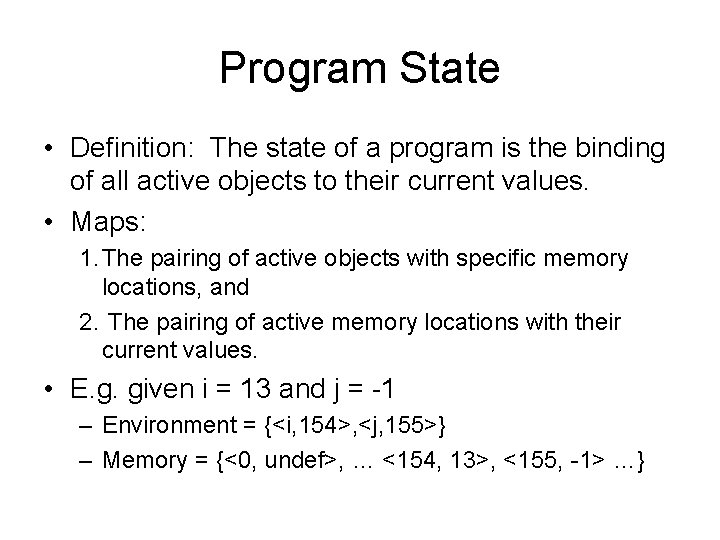Program State • Definition: The state of a program is the binding of all active objects to their current values. • Maps: 1. The pairing of active objects with specific memory locations, and 2. The pairing of active memory locations with their current values. • E. g. given i = 13 and j = -1 – Environment = {<i, 154>, <j, 155>} – Memory = {<0, undef>, … <154, 13>, <155, -1> …}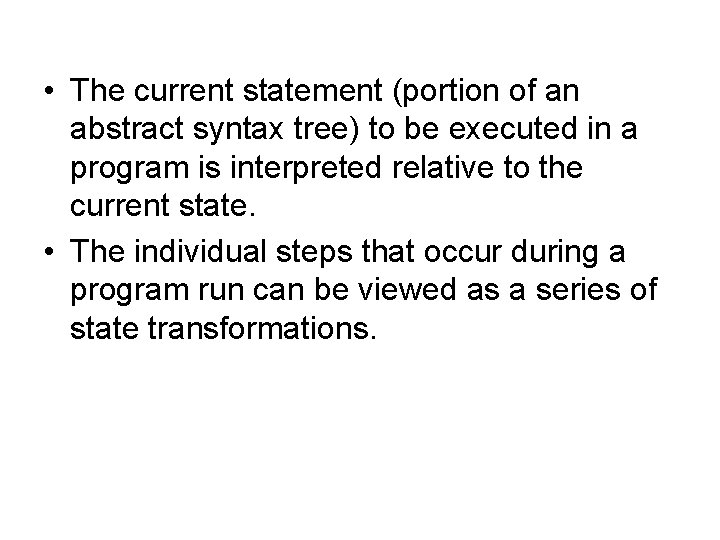• The current statement (portion of an abstract syntax tree) to be executed in a program is interpreted relative to the current state. • The individual steps that occur during a program run can be viewed as a series of state transformations.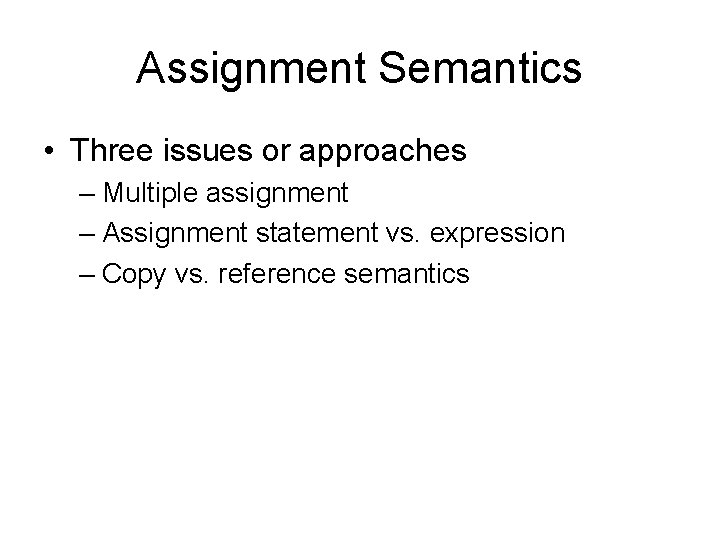Assignment Semantics • Three issues or approaches – Multiple assignment – Assignment statement vs. expression – Copy vs. reference semanticsMultiple Assignment • Example: • a = b = c = 0; • Sets all 3 variables to zero.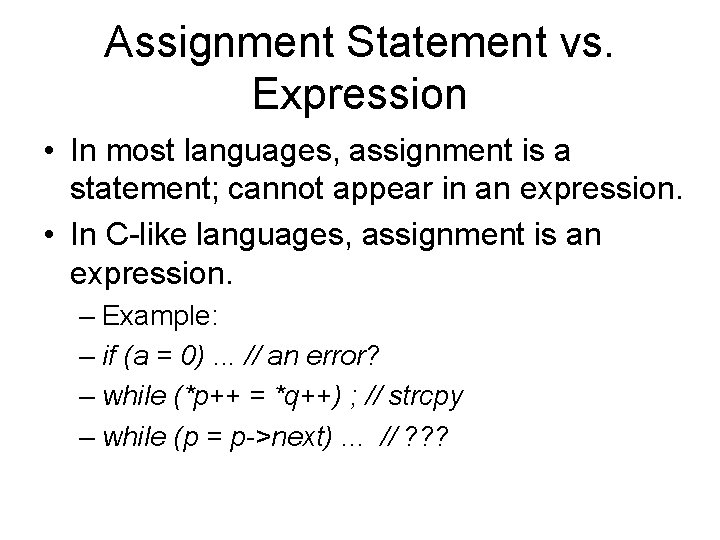Assignment Statement vs. Expression • In most languages, assignment is a statement; cannot appear in an expression. • In C-like languages, assignment is an expression. – Example: – if (a = 0). . . // an error? – while (*p++ = *q++) ; // strcpy – while (p = p->next). . . // ? ? ?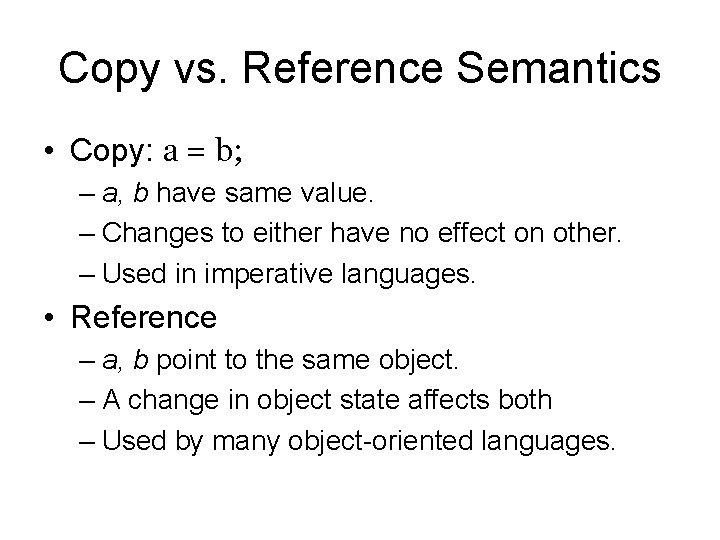Copy vs. Reference Semantics • Copy: a = b; – a, b have same value. – Changes to either have no effect on other. – Used in imperative languages. • Reference – a, b point to the same object. – A change in object state affects both – Used by many object-oriented languages.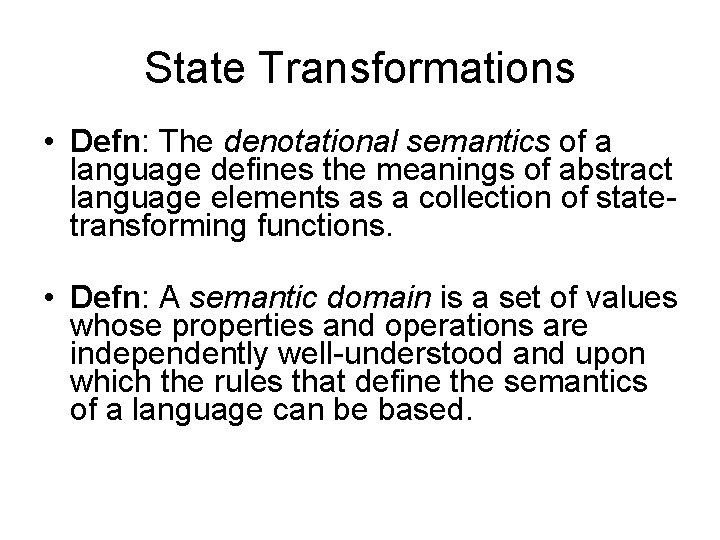State Transformations • Defn: The denotational semantics of a language defines the meanings of abstract language elements as a collection of statetransforming functions. • Defn: A semantic domain is a set of values whose properties and operations are independently well-understood and upon which the rules that define the semantics of a language can be based.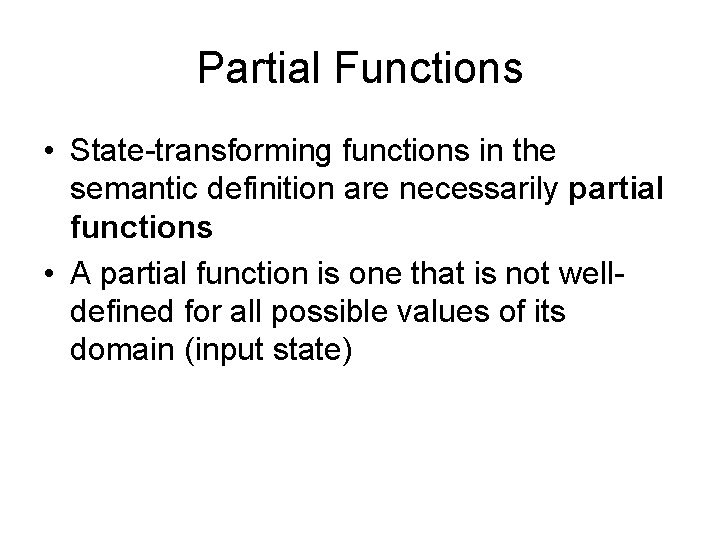Partial Functions • State-transforming functions in the semantic definition are necessarily partial functions • A partial function is one that is not welldefined for all possible values of its domain (input state)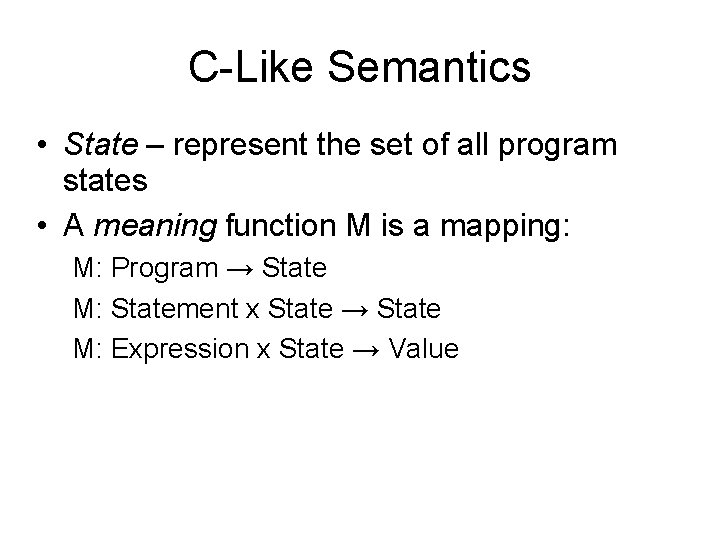C-Like Semantics • State – represent the set of all program states • A meaning function M is a mapping: M: Program → State M: Statement x State → State M: Expression x State → ValueMeaning Rule - Program • The meaning of a Program is defined to be the meaning of the body when given an initial state consisting of the variables of the decpart initialized to the undef value corresponding to the variable's type. State M (Program p) { // Program = Declarations decpart; Statement body return M(p. body, initial. State(p. decpart)); } public class State extends Hash. Map {. . . }State initial. State (Declarations d) { State state = new State( ); for (Declaration decl : d) state. put(decl. v, Value. mk. Value(decl. t)); return state; }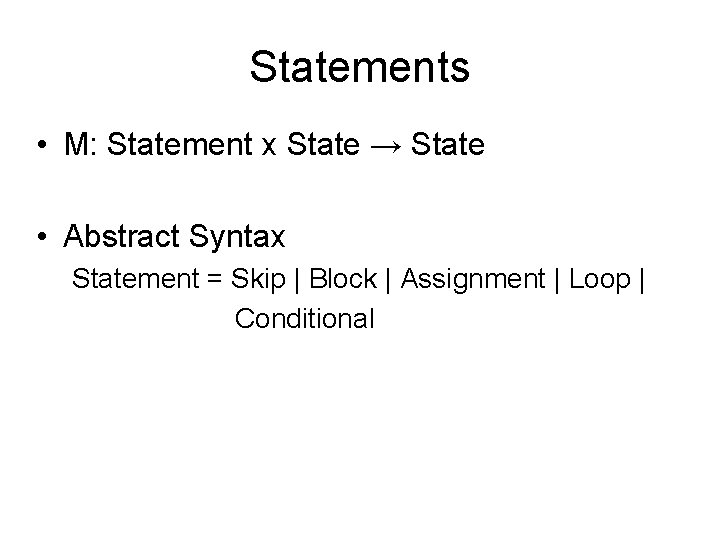Statements • M: Statement x State → State • Abstract Syntax Statement = Skip | Block | Assignment | Loop | Conditional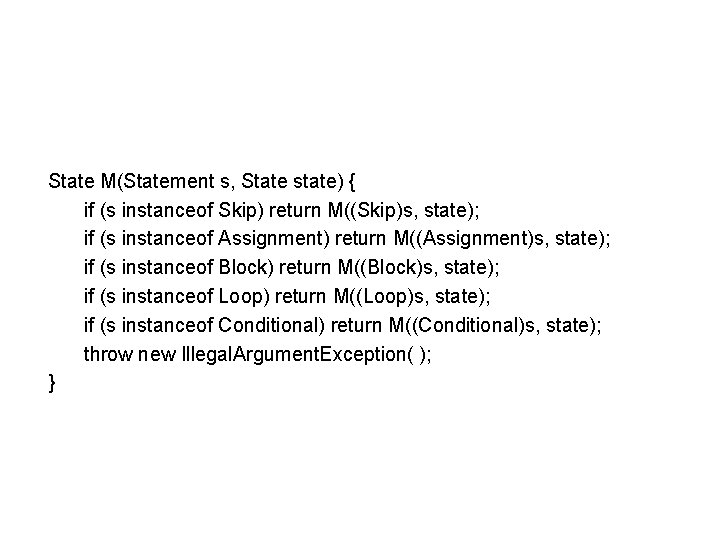State M(Statement s, State state) { if (s instanceof Skip) return M((Skip)s, state); if (s instanceof Assignment) return M((Assignment)s, state); if (s instanceof Block) return M((Block)s, state); if (s instanceof Loop) return M((Loop)s, state); if (s instanceof Conditional) return M((Conditional)s, state); throw new Illegal. Argument. Exception( ); }Meaning Rule - Skip • The meaning of a Skip is an identity function on the state; that is, the state is unchanged. State M(Skip s, State state) { return state; }Meaning Rule - Assignment • The meaning of an Assignment statement is the result of replacing the value of the target variable by the computed value of the source expression in the current state Assignment = Variable target; Expression sourceState M(Assignment a, State state) { return state. onion(a. target, M(a. source, state)); } // onion replaces the value of target in the map by the source // called onion because the symbol used is sometimes sigma σ to represent state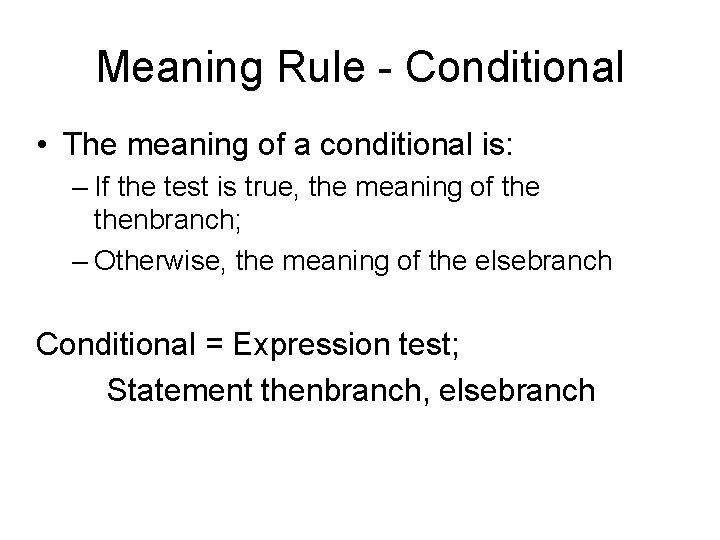Meaning Rule - Conditional • The meaning of a conditional is: – If the test is true, the meaning of thenbranch; – Otherwise, the meaning of the elsebranch Conditional = Expression test; Statement thenbranch, elsebranch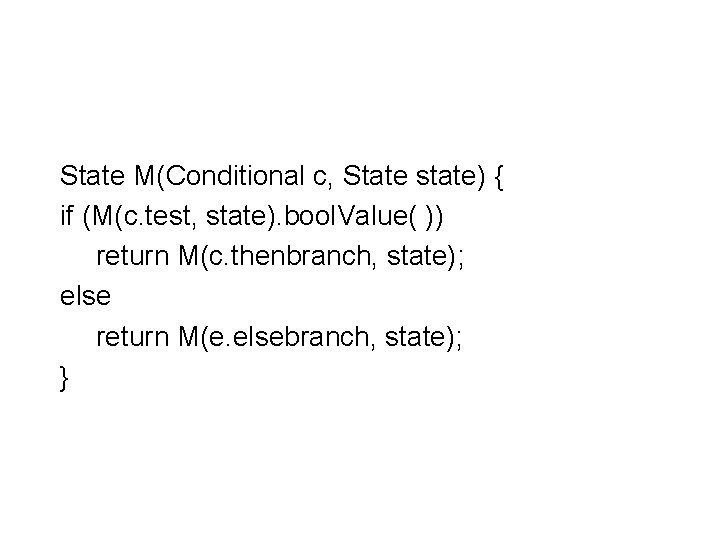State M(Conditional c, State state) { if (M(c. test, state). bool. Value( )) return M(c. thenbranch, state); else return M(e. elsebranch, state); }Expressions • M: Expression x State → Value • • • Expression = Variable | Value | Binary | Unary Binary = Binary. Op op; Expression term 1, term 2 Unary = Unary. Op op; Expression term Variable = String id Value = Int. Value | Bool. Value | Char. Value | Float. Value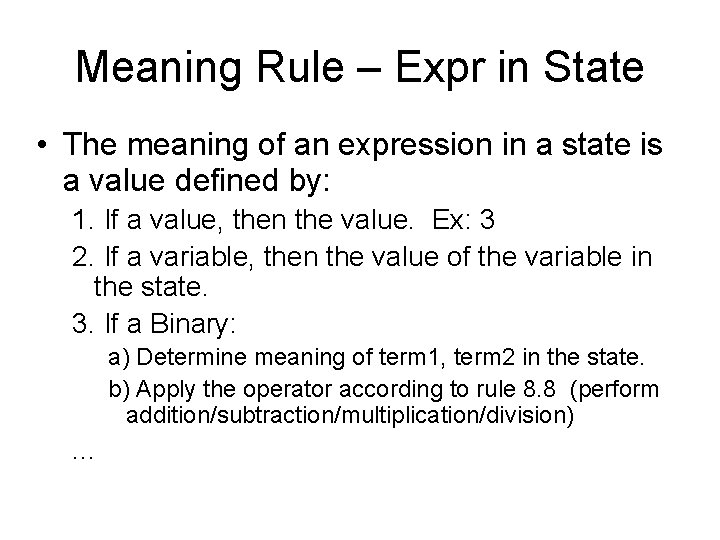Meaning Rule – Expr in State • The meaning of an expression in a state is a value defined by: 1. If a value, then the value. Ex: 3 2. If a variable, then the value of the variable in the state. 3. If a Binary: a) Determine meaning of term 1, term 2 in the state. b) Apply the operator according to rule 8. 8 (perform addition/subtraction/multiplication/division) . . .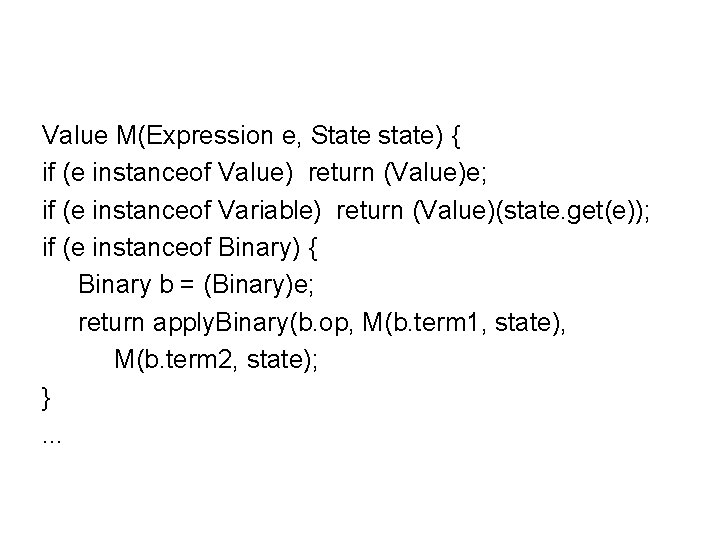Value M(Expression e, State state) { if (e instanceof Value) return (Value)e; if (e instanceof Variable) return (Value)(state. get(e)); if (e instanceof Binary) { Binary b = (Binary)e; return apply. Binary(b. op, M(b. term 1, state), M(b. term 2, state); }. . .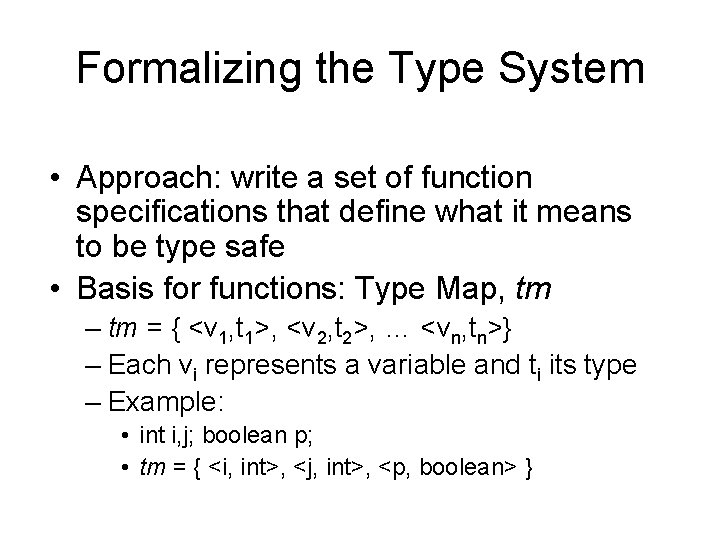Formalizing the Type System • Approach: write a set of function specifications that define what it means to be type safe • Basis for functions: Type Map, tm – tm = { <v 1, t 1>, <v 2, t 2>, … <vn, tn>} – Each vi represents a variable and ti its type – Example: • int i, j; boolean p; • tm = { <i, int>, <j, int>, <p, boolean> }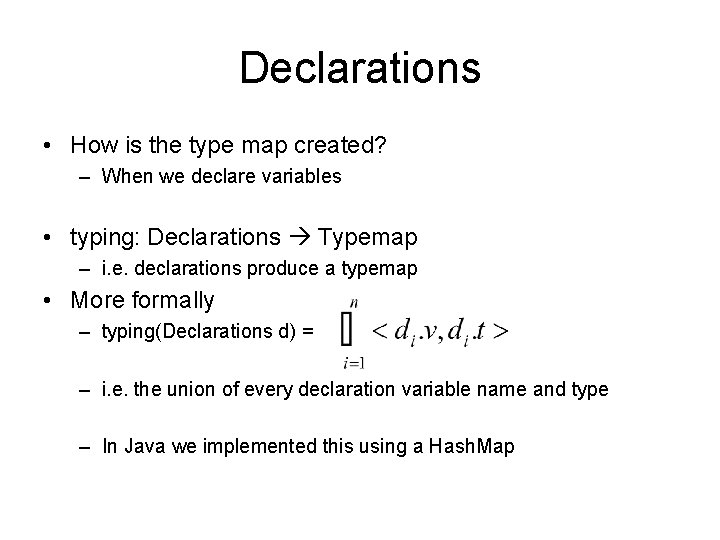Declarations • How is the type map created? – When we declare variables • typing: Declarations Typemap – i. e. declarations produce a typemap • More formally – typing(Declarations d) = – i. e. the union of every declaration variable name and type – In Java we implemented this using a Hash. MapSemantic Domains and States • Beyond types, we must determine semantically what the syntax means • Semantic Domains are a formalism we will use – Environment, γ = set of pairs of variables and memory locations • γ = {<i, 100>, <j, 101>} for i at Addr 100, j at Addr 101 – Memory, μ = set of pairs of memory locations and the value stored there • μ = {<100, 10> , <101, 50>} for Mem(100)=10, Mem(101)=50 – State of the program, σ = set of pairs of active variables and their current values • σ = {<i, 10>, <j, 50>} for i=10, j=50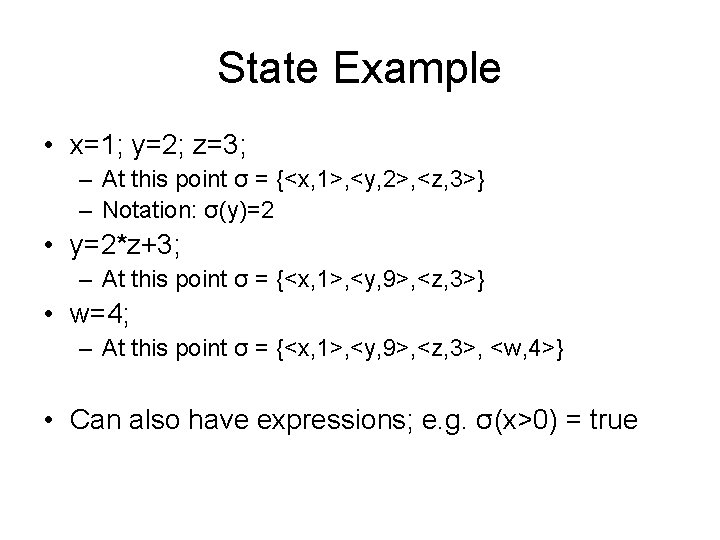State Example • x=1; y=2; z=3; – At this point σ = {<x, 1>, <y, 2>, <z, 3>} – Notation: σ(y)=2 • y=2*z+3; – At this point σ = {<x, 1>, <y, 9>, <z, 3>} • w=4; – At this point σ = {<x, 1>, <y, 9>, <z, 3>, <w, 4>} • Can also have expressions; e. g. σ(x>0) = true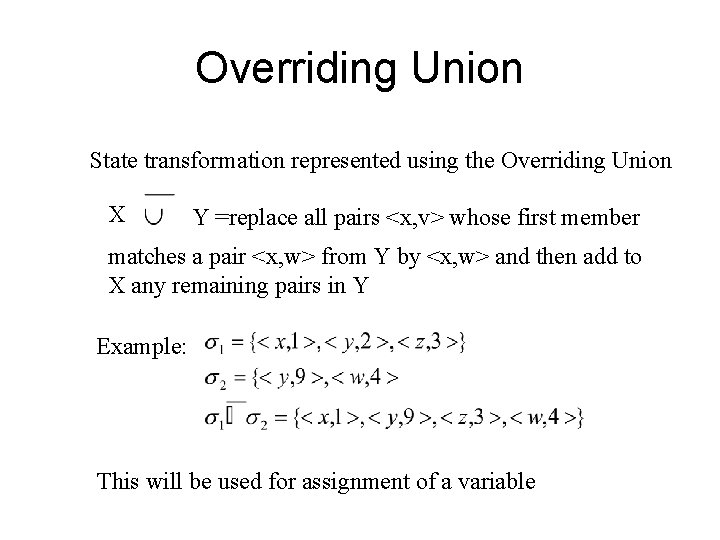Overriding Union State transformation represented using the Overriding Union X Y =replace all pairs <x, v> whose first member matches a pair <x, w> from Y by <x, w> and then add to X any remaining pairs in Y Example: This will be used for assignment of a variableDenotational Semantics • Meaning function – Input: abstract class, current state – Output: new state Let’s revisit our Meaning Rules and redefine them using our more Formal Denotational SemanticsDenotational Semantics Meaning of a program: produce final state This is just the meaning of the body in an initial state Java implementation: State M (Program p) { // Program = Declarations decpart; Statement body return M(p. body, initial. State(p. decpart)); } public class State extends Hash. Map {. . . }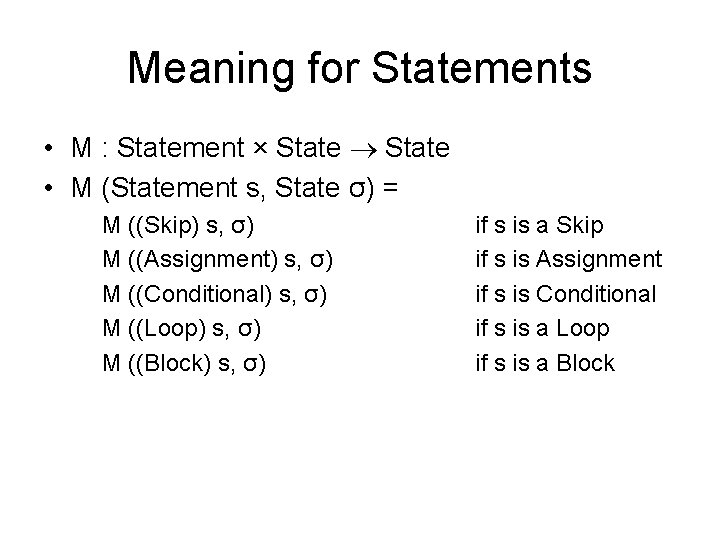Meaning for Statements • M : Statement × State • M (Statement s, State σ) = M ((Skip) s, σ) M ((Assignment) s, σ) M ((Conditional) s, σ) M ((Loop) s, σ) M ((Block) s, σ) if s is a Skip if s is Assignment if s is Conditional if s is a Loop if s is a Block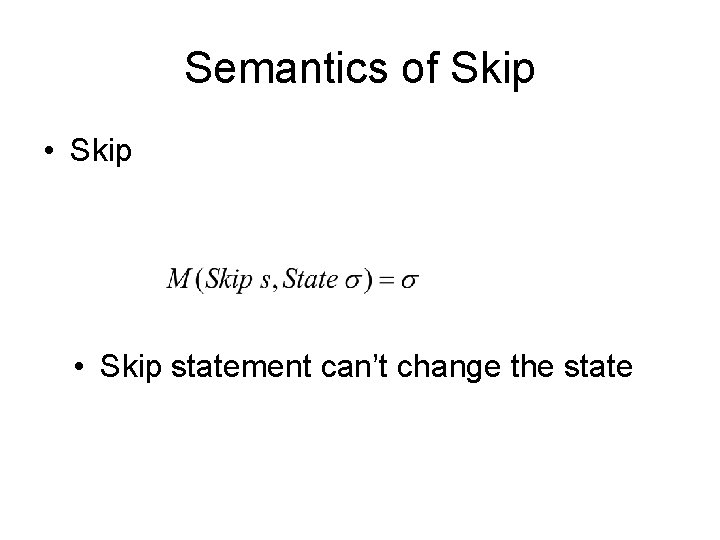Semantics of Skip • Skip statement can’t change the stateSemantics of Assignment • Evaluate expression and assign to var • Examples of: x=a+b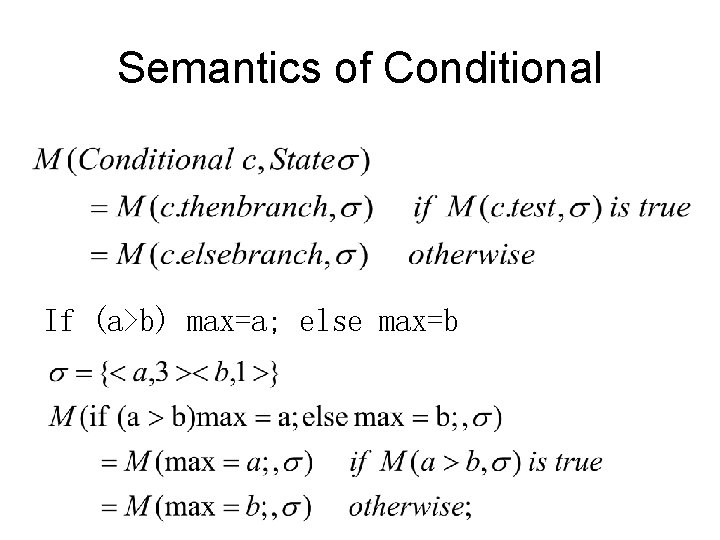Semantics of Conditional If (a>b) max=a; else max=bConditional, continuedSemantics of Block • Block is just a sequence of statements • Example for Block b: fact = fact * i; i = i – 1;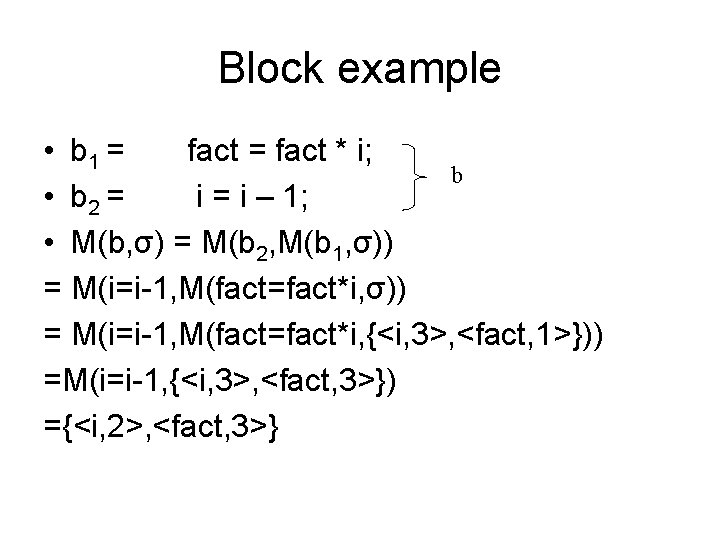Block example • b 1 = fact * i; b • b 2 = i – 1; • M(b, σ) = M(b 2, M(b 1, σ)) = M(i=i-1, M(fact=fact*i, {<i, 3>, <fact, 1>})) =M(i=i-1, {<i, 3>, <fact, 3>}) ={<i, 2>, <fact, 3>}Semantics of Loop • Loop = Expression test; Statement body • Recursive definitionLoop Example • Initial state σ={<N, 3>} fact=1; i=N; while (i>1) { fact = fact * i; i = i -1; } After first two statements, σ = {<fact, 1>, <N, 3>, <i, 3>}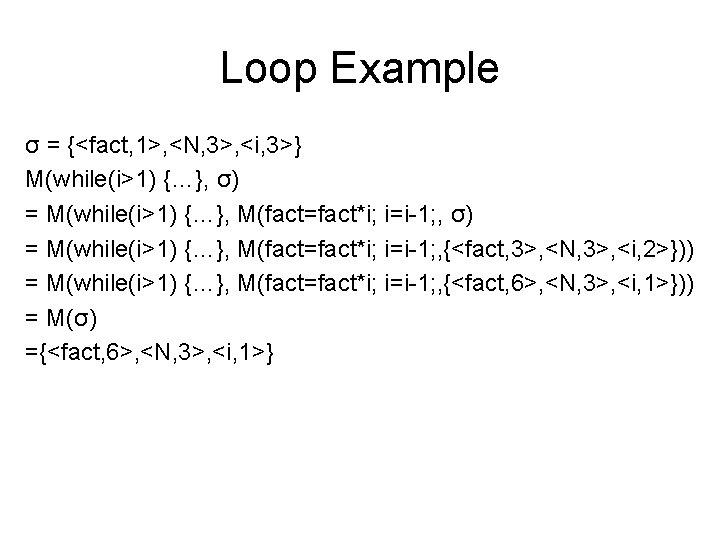Loop Example σ = {<fact, 1>, <N, 3>, <i, 3>} M(while(i>1) {…}, σ) = M(while(i>1) {…}, M(fact=fact*i; i=i-1; , {<fact, 3>, <N, 3>, <i, 2>})) = M(while(i>1) {…}, M(fact=fact*i; i=i-1; , {<fact, 6>, <N, 3>, <i, 1>})) = M(σ) ={<fact, 6>, <N, 3>, <i, 1>}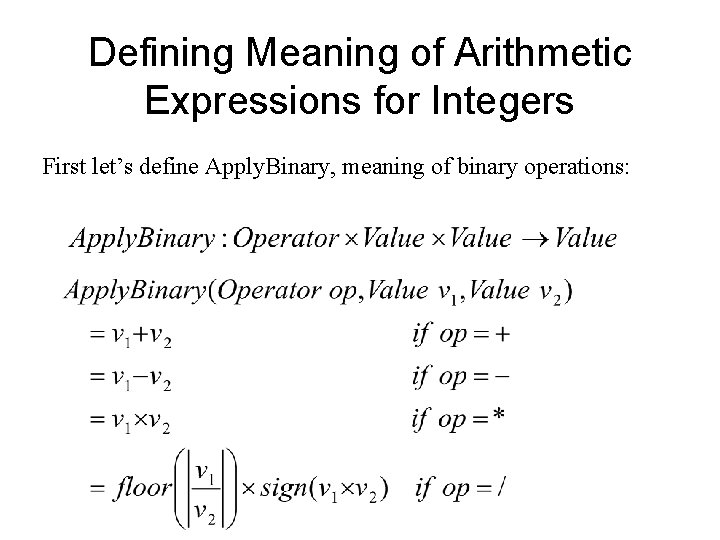Defining Meaning of Arithmetic Expressions for Integers First let’s define Apply. Binary, meaning of binary operations: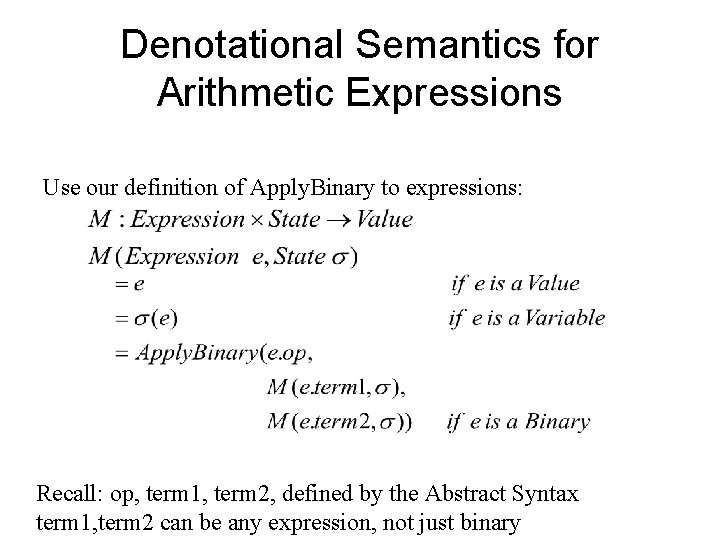Denotational Semantics for Arithmetic Expressions Use our definition of Apply. Binary to expressions: Recall: op, term 1, term 2, defined by the Abstract Syntax term 1, term 2 can be any expression, not just binary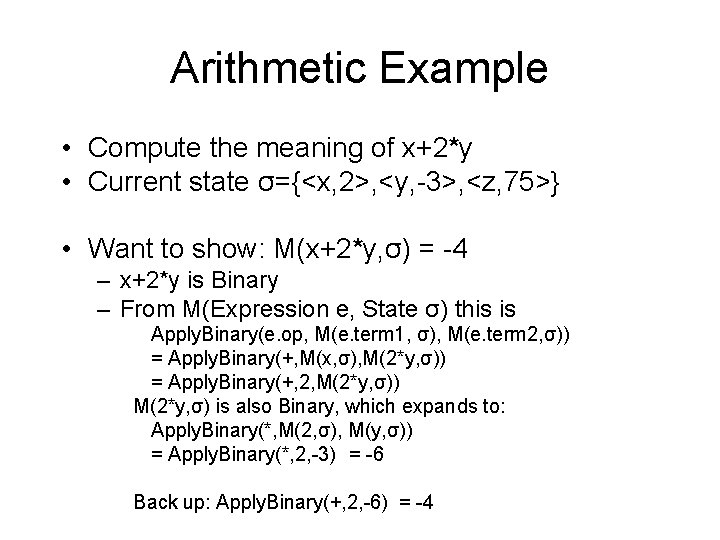Arithmetic Example • Compute the meaning of x+2*y • Current state σ={<x, 2>, <y, -3>, <z, 75>} • Want to show: M(x+2*y, σ) = -4 – x+2*y is Binary – From M(Expression e, State σ) this is Apply. Binary(e. op, M(e. term 1, σ), M(e. term 2, σ)) = Apply. Binary(+, M(x, σ), M(2*y, σ)) = Apply. Binary(+, 2, M(2*y, σ)) M(2*y, σ) is also Binary, which expands to: Apply. Binary(*, M(2, σ), M(y, σ)) = Apply. Binary(*, 2, -3) = -6 Back up: Apply. Binary(+, 2, -6) = -4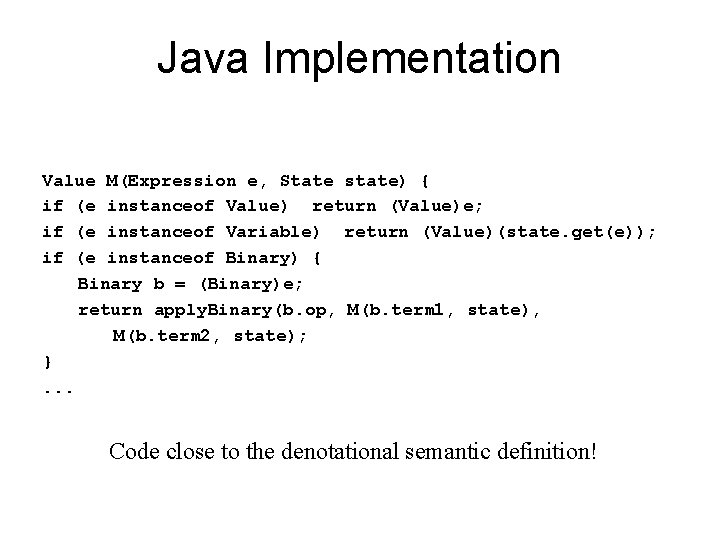Java Implementation Value M(Expression e, State state) { if (e instanceof Value) return (Value)e; if (e instanceof Variable) return (Value)(state. get(e)); if (e instanceof Binary) { Binary b = (Binary)e; return apply. Binary(b. op, M(b. term 1, state), M(b. term 2, state); }. . . Code close to the denotational semantic definition!